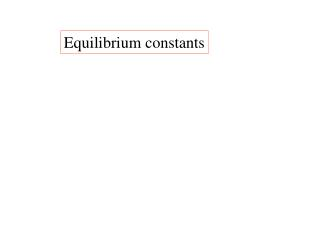DownloadDownload PresentationEquilibrium constants

# Equilibrium constants

Télécharger la présentation## Equilibrium constants

- - - - - - - - - - - - - - - - - - - - - - - - - - - E N D - - - - - - - - - - - - - - - - - - - - - - - - - - -
##### Presentation Transcript

1. Equilibrium constants

2. Equilibrium constants PCcPDd K = PAaPBb [C]c[D]d K = [A]a[B]b

3. Equilibrium constants PCcPDd K = PAaPBb [C]c[D]d K = [A]a[B]b [solid] = 1

4. Equilibrium of solids and solutions

5. Equilibrium of solids and solutions NaCl(s) + H2O(l)

6. Equilibrium of solids and solutions NaCl(s) + H2O(l) Na+(aq) + Cl-(aq) To remove an ion from the crystal lattice, the solvating interactions must be stronger than the lattice interactions.

7. Equilibrium of solids and solutions NaCl(s) + H2O(l) Na+(aq) + Cl-(aq) Solution must be saturated for this equilibrium to take place.

8. Dynamic equilibrium

9. Equilibrium for dissolution-precipitation reaction: I2(s) + CCl4(l) I2(CCl4)

10. Equilibrium for dissolution-precipitation reaction: I2(s) + CCl4(l) I2(CCl4) K [I2]CCl = 4

11. Equilibrium for dissolution-precipitation reaction: I2(s) + CCl4(l) I2(CCl4) [I2]CCl K = 4 Molarity of saturated solution = K

12. [I2]CCl K = 4 K will vary when conditions are changed based on Le Chatelier’s Principle.

13. [I2]CCl K = 4 If solution of a material is exothermic, increasing the temperature will decrease K. A(s) A(sol)

14. [I2]CCl K = 4 If solution of a material is endothermic, increasing the temperature will increase K. A(s) A(sol)

15. Kinetics (reaction rates) must be considered.

16. The value of K is not an indicator of howlong it takes to attain equilibrium.

17. A K = 5 does not guarantee a 5 M solution in a few minutes.

18. Not all solutions are ‘ideal’

19. Not all solutions are ‘ideal’ Ideal Solution: Widely separated species (ions or molecules) that do not interact.

20. CsCl(s) Cs+(aq) + Cl-(aq)

21. CsCl(s) Cs+(aq) + Cl-(aq) As the concentration of ions increases, Cs+to Cl- distances decrease.

22. CsCl(s) Cs+(aq) + Cl-(aq) As the concentration of ions increases, Cs+ to Cl- distances decrease. Cs+…Cl- Cs+…Cl- Cl-…Cs+

23. CsCl(s) Cs+(aq) + Cl-(aq) Cs+…Cl- Cs+…Cl- Cl-…Cs+ Ion pairing may occur before equilibrium.

24. CsCl(s) Cs+(aq) + Cl-(aq) Cs+…Cl- Cs+…Cl- Cl-…Cs+ Ion pairing may occur before equilibrium. These solutions are non-ideal.

25. CsCl(s) Cs+(aq) + Cl-(aq) Salts of low solubilities allow the study of solutions that are essentially ideal.

26. CsCl(s) Cs+(aq) + Cl-(aq) Salts of low solubilities allow the study of solutions that are essentially ideal. A saturated solution of 0.1 or less is a sign of low solubility in a salt.

27. Solubility product

28. Solubility product AgCl(s) Ag+(aq) + Cl-(aq)

29. Solubility product Same form as equilibrium constant AgCl(s) Ag+(aq) + Cl-(aq)

30. Solubility product AgCl(s) Ag+(aq) + Cl-(aq) [Ag+][Cl-] [AgCl]

31. Solubility product AgCl(s) Ag+(aq) + Cl-(aq) [Ag+][Cl-] = Ksp 1

32. Solubility product AgCl(s) Ag+(aq) + Cl-(aq) [Ag+][Cl-] = Ksp

33. Solubility product AgCl(s) Ag+(aq) + Cl-(aq) [Ag+][Cl-] = Ksp 25oC Ksp = 1.6 x 10-10

34. Solubility product AgCl(s) Ag+(aq) + Cl-(aq) [Ag+][Cl-] = Ksp 25oC Ksp = 1.6 x 10-10 [Ag+] = [Cl-] = y

35. Solubility product AgCl(s) Ag+(aq) + Cl-(aq) [Ag+][Cl-] = Ksp 25oC Ksp = 1.6 x 10-10 [Ag+] = [Cl-] = y y2 = Ksp = 1.6 x 10-10

36. Solubility product AgCl(s) Ag+(aq) + Cl-(aq) [Ag+][Cl-] = Ksp y2 = Ksp = 1.6 x 10-10 [Ag+] = [Cl-] = 1.26 x 10-5 M

37. Solubility product AgCl(s) Ag+(aq) + Cl-(aq) [Ag+][Cl-] = Ksp y2 = Ksp = 1.6 x 10-10 1.8 x 10-3 g/L [Ag+] = [Cl-] = 1.26 x 10-5 M

38. BaF2(s) Ba2+(aq) + 2 F-(aq)

39. BaF2(s) Ba2+(aq) + 2 F-(aq) [Ba2+][F-]2 = Ksp

40. Fe3+(aq) + 3 OH-(aq) Fe(OH)3

41. Fe3+(aq) + 3 OH-(aq) Fe(OH)3 [Fe3+][OH-]3 = 1.1 x 10-36

42. Fe3+(aq) + 3 OH-(aq) Fe(OH)3 [Fe3+][OH-]3 = 1.1 x 10-36 For every Fe3+ that goes into solution, 3 OH- go into solution.

43. Fe3+(aq) + 3 OH-(aq) Fe(OH)3 [Fe3+][OH-]3 = 1.1 x 10-36 [y][3y]3 = 1.1 x 10-36

44. Fe3+(aq) + 3 OH-(aq) Fe(OH)3 [Fe3+][OH-]3 = 1.1 x 10-36 [y][3y]3 = 1.1 x 10-36 If there is another source of OH- (NaOH) that provides a higher [OH-] then that is the value of [OH-] to be used.